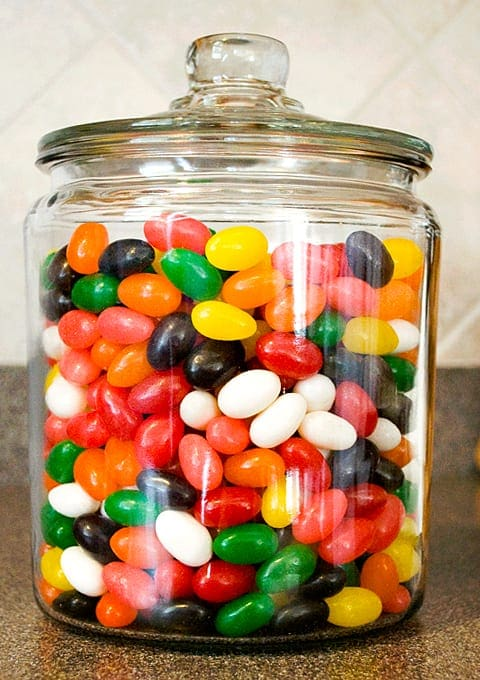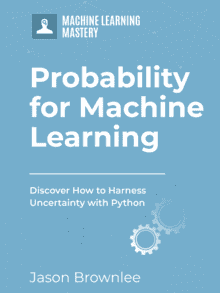# Do Not Use Random Guessing As Your Baseline Classifier

I recently received the following question via email:

Hi Jason, quick question. A case of class imbalance: 90 cases of thumbs up 10 cases of thumbs down. How would we calculate random guessing accuracy in this case?

We can answer this question using some basic probability (I opened excel and typed in some numbers).

Kick-start your project with my new book Probability for Machine Learning, including step-by-step tutorials and the Python source code files for all examples.

Let’s get started.

Note, for a more detailed tutorial on this topic, see:Don’t Use Random Guessing As Your Baseline Classifier
Photo by cbgrfx123, some rights reserved.

Let’s say the split is 90%-10% for class 0 and class 1. Let’s also say that you will guess randomly using the same ratio.

The theoretical accuracy of random guessing on a two-classification problem is:

We can test this on our example 90%-10% split:

To check the math, you can plug-in a 50%-50% split of your data and it matches your intuition:

If we look on Google, we find a similar question on Cross Validated “What is the chance level accuracy in unbalanced classification problems?” with an almost identical answer. Again, a nice confirmation.

Interesting, but there is an important takeaway point from all of this.

### Want to Learn Probability for Machine Learning

Take my free 7-day email crash course now (with sample code).

Click to sign-up and also get a free PDF Ebook version of the course.

## Don’t Use Random Guessing As A Baseline

If you are looking for a classifier to use as a baseline accuracy, don’t use random guessing.

There is a classifier called Zero Rule (or 0R or ZeroR for short). It is the simplest rule you can use on a classification problem and it simply predicts the majority class in your dataset (e.g. the mode).

In the example above with a 90%-10% for class 0 and class 1 it would predict class 0 for every prediction and achieve an accuracy of 90%. This is 8% better than the theoretical maximum using random guessing.

Use the Zero Rule method as a baseline.

Also, in imbalanced classification problems like this, you should use metrics other than Accuracy such as Kappa or Area under ROC Curve.

For more on working with imbalanced classification problems see the post:

## Get a Handle on Probability for Machine Learning!#### Develop Your Understanding of Probability

...with just a few lines of python code

Discover how in my new Ebook:
Probability for Machine Learning

It provides self-study tutorials and end-to-end projects on:
Bayes Theorem, Bayesian Optimization, Distributions, Maximum Likelihood, Cross-Entropy, Calibrating Models
and much more...

### 11 Responses to Do Not Use Random Guessing As Your Baseline Classifier

1.Atanu Maity August 5, 2019 at 12:55 pm #

Hi Jason, very useful article. Just curious to know, Suppose in my dataset, the target label ration is 1:4 (yes vs no). Then what should be the accuracy of a random classification model, which I may choose as my baseline?

•Jason Brownlee August 5, 2019 at 2:05 pm #

If it is binary classification, the baseline performance would be 75% accuracy.

•Atanu Maity August 5, 2019 at 2:33 pm #

Thanks for the reply. Can you please brief the calculation, how you did that? I just want to learn

•Atanu Maity August 5, 2019 at 2:57 pm #

As per the ZeroR rule, the baseline accuracy should be 80%, as the Y/N ratio is 1:4. Then how you calculated it as 75%?

•Jason Brownlee August 6, 2019 at 6:28 am #

If the ratio is 1 to 4, then in 100 samples, 75 are one class and 25 another.

Predicting the major class for all records means that 75 of those examples would be correct, 25 would be incorrect, meaning accuracy would be 75%.

•Atanu Maity August 6, 2019 at 1:05 pm #

“If the ratio is 1 to 4, then in 100 samples, 75 are one class and 25 another.”-

Shouldn’t it be 80 are one class and 20 another, in 100 samples?

20:80 = 1:4 but 25:75 = 1:3

•Jason Brownlee August 6, 2019 at 2:08 pm #

I was taking 1:4 as 1/4:

1/4 = 0.25
25 * 4 = 100

If you mean 1 to 4, then you are right, the ratio is 1/5 or 80% accuracy.

2.Michalis May 6, 2020 at 1:35 am #

Thank for the great post! So what if we have a multi-class problem? What best naive ‘baseline’ would you recommend?

•Jason Brownlee May 6, 2020 at 6:28 am #

Good question, it depends on the metric you use, see this:
https://machinelearningmastery.mystagingwebsite.com/naive-classifiers-imbalanced-classification-metrics/

•Michalis May 11, 2020 at 5:03 am #

Thank you!! That is very insightful!

•Jason Brownlee May 11, 2020 at 6:10 am #

You’re welcome.